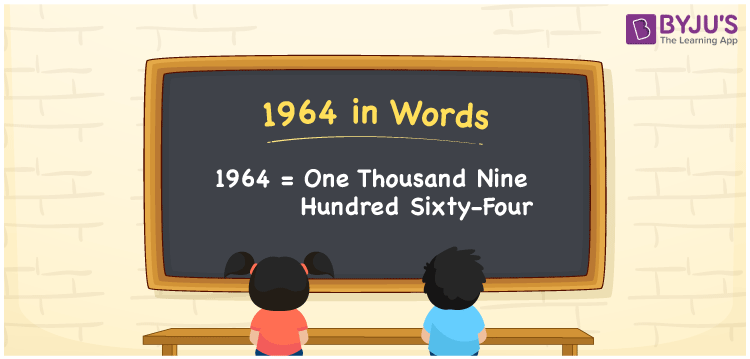# 1964 in Words

1964 in words can be written as One Thousand Nine Hundred Sixty-Four. If the current bill for a month is Rs. 1964, then you can say that “The current bill for a month is One Thousand Nine Hundred Sixty-Four Rupees”. Get to know the place value of each digit in 1964 in detail in this article. So, the number 1964 can be read as “One Thousand Nine Hundred Sixty-Four” in words.

 1964 in words One Thousand Nine Hundred Sixty-Four One Thousand Nine Hundred Sixty-Four in Numbers 1964

## 1964 in English Words## How to Write 1964 in Words?

The concept of place value in 1964 can be learnt effectively from the table provided below. Using it, writing numbers in words will be easy.

 Thousands Hundreds Tens Ones 1 9 6 4

1964 in expanded form can be written as:

1 x Thousand + 9 × Hundred + 6 × Ten + 4 × One

= 1 x 1000 + 9 x 100 + 6 x 10 + 4 x 1

= 1000 + 900 + 60 + 4

= 1964

= One Thousand Nine Hundred Sixty-Four

Hence, 1964 in words is written as One Thousand Nine Hundred Sixty-Four.

1964 is a natural number that precedes 1965 and succeeds 1963.

1964 in words – One Thousand Nine Hundred Sixty-Four

Is 1964 an odd number? – No

Is 1964 an even number? – Yes

Is 1964 a perfect square number? – No

Is 1964 a perfect cube number? – No

Is 1964 a prime number? – No

Is 1964 a composite number? – Yes

## Frequently Asked Questions on 1964 in Words

Q1

### How to write 1964 in words?

1964 can be written in words as “One Thousand Nine Hundred Sixty-Four”.
Q2

### Write One Thousand Nine Hundred Sixty-Four in numbers.

One Thousand Nine Hundred Sixty-Four in numbers can be written as 1964.
Q3

### Is 1964 an even number?

1964 is an even number as it is completely divisible by 2.